The CAS procedure (PROC CAS) enables us to interact with SAS Cloud Analytic Services (CAS) from the SAS client based on the CASL (the scripting language of CAS) specification. CASL supports a variety of data types including INT, DOUBLE, STRING, TABLE, LIST, BLOB, and others. The result of a CAS action could be any of the data types mentioned above. In this article, we will use the CAS procedure to explore techniques to interact with the results produced from CAS actions.

PROC CAS enables us to run CAS actions in SAS Viya; CAS actions are at the heart of how the CAS server receives requests, performs the relevant tasks and returns results back to the user. CAS actions representing common functionality constitute CAS action sets.  Consider the CAS action set TABLE. Within the TABLE action set, we will currently find 24 different actions relevant to various tasks that can be performed on a table. For example, the COLUMNINFO action within the TABLE action set will inform the user of the contents of a CAS in-memory table, including column names, lengths, data types, and so on. Let’s take a look at the following code to understand how this works:

```proc cas; table.columnInfo / table='HMEQ'; simple.summary / table={name='HMEQ' groupby='BAD'}; run;```

In the code above, the table called ‘HMEQ’ is a distributed in-memory CAS table that contains data about applicants who were granted credit for a certain home equity loan. The categorical binary-valued variable ‘BAD’ identifies a client who has either defaulted or repaid their home equity loan. Since PROC CAS is an interactive procedure, we can invoke multiple statements within a single run block. In the code above we have executed the COLUMNINFO action from the TABLE actionset and the SUMMARY action from the SIMPLE action set within the same run block. Notice that we are able to obtain a summary statistic of all the interval variables in the dataset grouped by the binary variable ‘BAD’ without having to first sort the data by that variable. Below is a snapshot of the resulting output.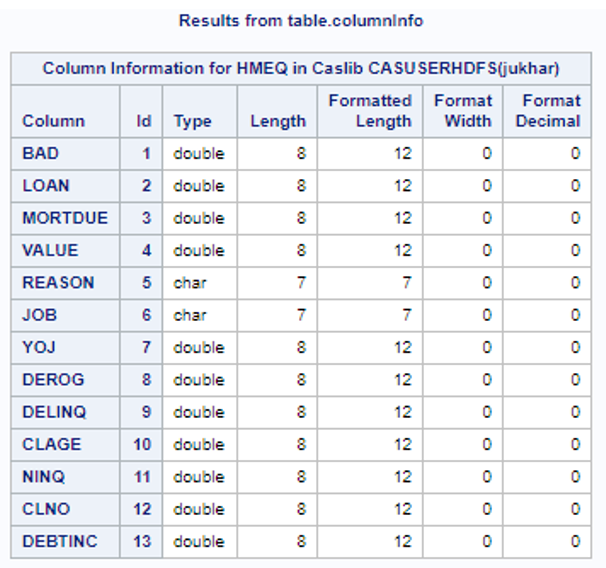Fig1: Output from table.columninfo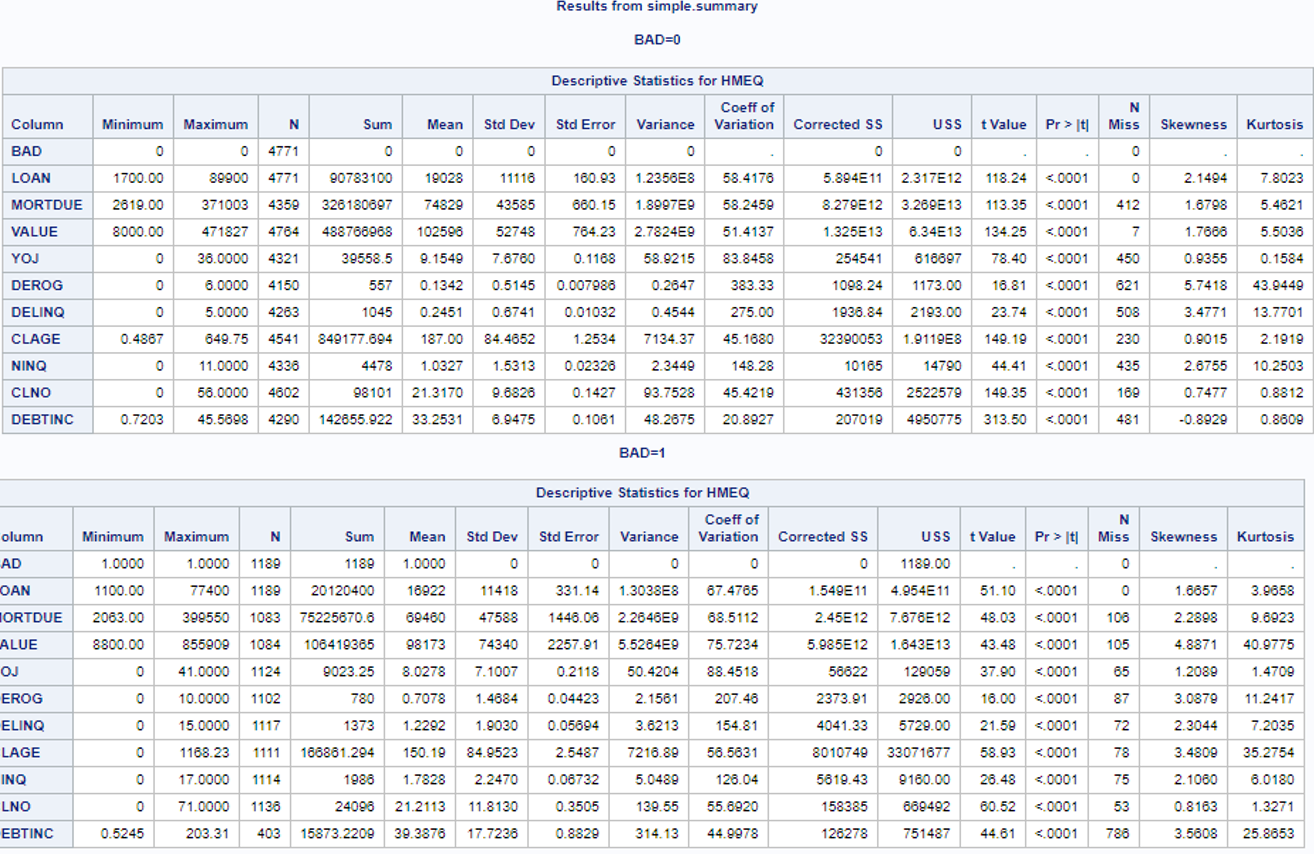Fig 2: Output from executing the summary actionset with a BY group option

Let’s dig into this a little deeper by considering the following statements:

```proc cas; simple.summary result=S /table={name='hmeq'}; describe S; run;```

In the code snippet above, the result of the summary action is returned to the user in a variable ‘S’ which is a dictionary. How do we know? To find that, we have invoked the DESCRIBE statement to help us understand exactly what the form of this result, S, is. The DESCRIBE statement is used to display the data type of a variable. Let’s take a look at the log file: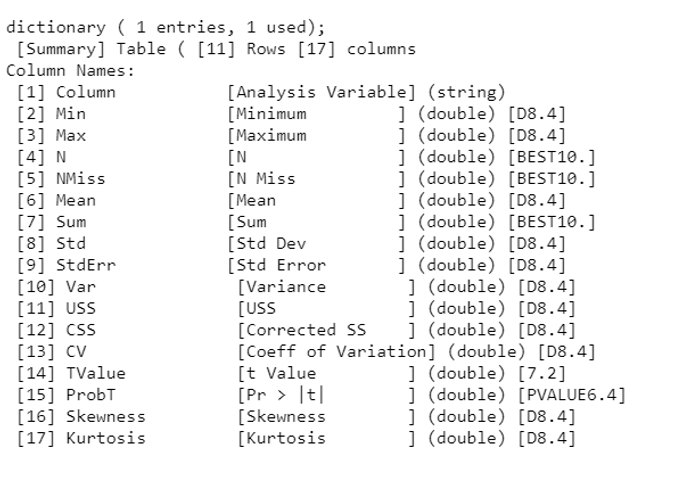The log file informs the user that ‘S’ is a dictionary with one entry of type table named “Summary.” In the above example the column names and the attributes are shown on the log file. If we want to print the entire summary table or a subset, we would use the code below:

```proc cas; simple.summary result=S /table={name='hmeq'}; print s[“Summary”]; print s[“summary”, 3:5]; run;```

The first PRINT statement will fetch the entire summary table; the second PRINT statement will fetch rows 3 through 5. We can also use WHERE expression processing to create a new table with rows that match the WHERE expression. The output of the second PRINT statements above are shown in the figure below: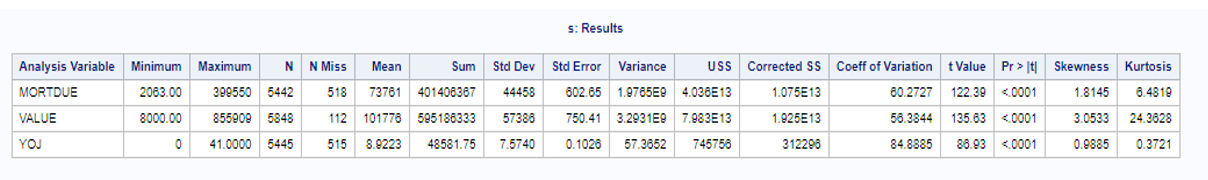The result of an action could also be more complex in nature; it could be a dictionary containing dictionaries, arrays, and lists, or the result could be a list containing lists and arrays and tables etc. Therefore, it is important to understand these concepts through some simple cases. Let’s consider another example where the result is slightly more complex.

```proc cas; simple.summary result=s /table={name='hmeq', groupby='bad'}; describe s; print s["ByGroup1.Summary", 3:5]; run;```

In the example above, we are executing a summary using a BY group on a binary variable. The log shows that the result in this case is a dictionary with three entries, all of which are tables. Below is a snapshot of the log file as well as the output of PRINT statement looking at the summary for the first BY group for row 3 through 5.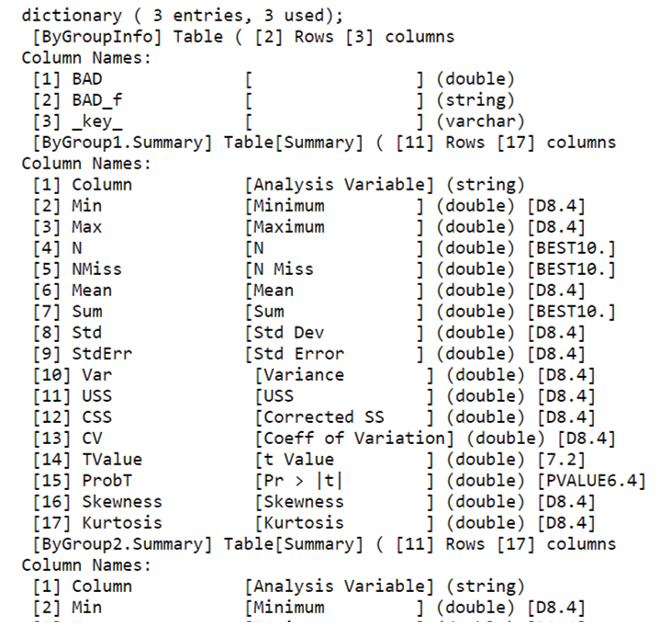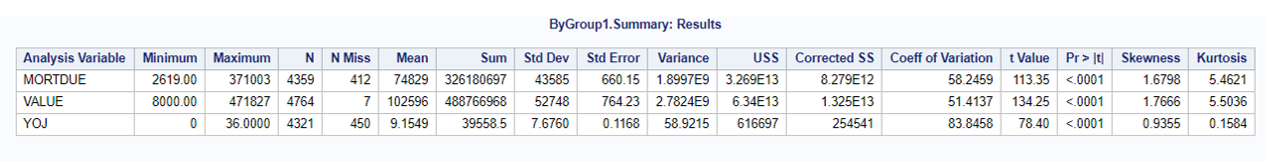If we are interested in saving the output of the summary action as a SAS data set (sas7bdat file), we will execute the SAVERESULT statement. The code below saves the summary statistics of the first BY group in the work library as a SAS dataset.

```proc cas; simple.summary result=s /table={name='hmeq', groupby='bad'}; describe s; print s["ByGroup1.Summary", 3:5]; saveresult s["ByGroup1.Summary"] dataout=work.data; run;```

A helpful function we can often use is findtable. This function will search the given value for the first table it finds.

```proc cas; simple.summary result=s /table={name='hmeq'}; val = findtable(s); saveresult val dataout=work.summary; run;```

In the example above, I used findtable to locate the first table produced within the dictionary, S, and save it under ‘Val,’ which is then directly invoked with SAVERESULT statement to save the output as a SAS dataset.

Finally, we also have the option to save the output of a CAS action in another CAS Table. The table summary1 in the code below is an in-memory CAS table that contains the output of the summary action. The code and output are shown below:

```proc cas; simple.summary /table={name='hmeq'} casout={name='mylib.summary1'}; fetch /table={name='mylib.summary1'}; run;```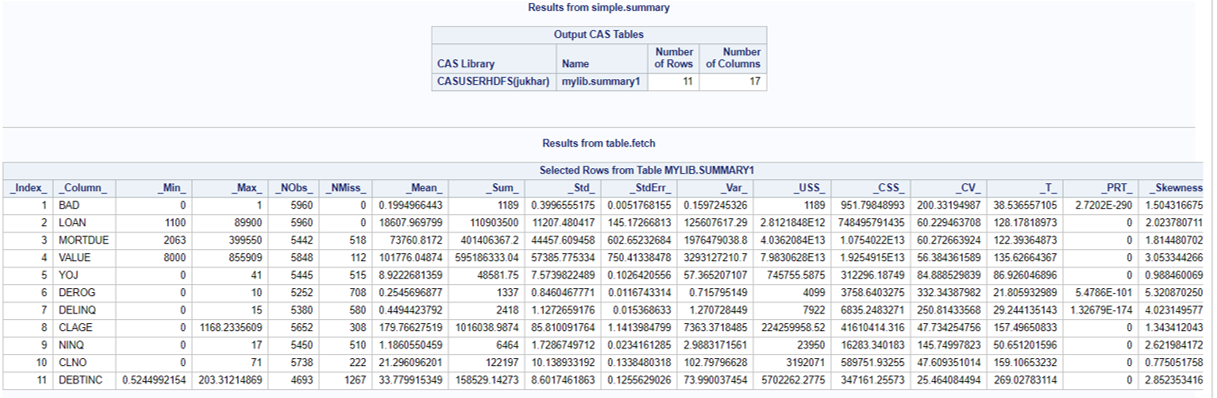In this post, we saw several ways of interacting with the results of a CAS action using the CAS procedure. Depending on what our end goal is, we can use any of these options to either view the results or save the data for further processing.

Interacting with the results of PROC CAS was published on SAS Users.